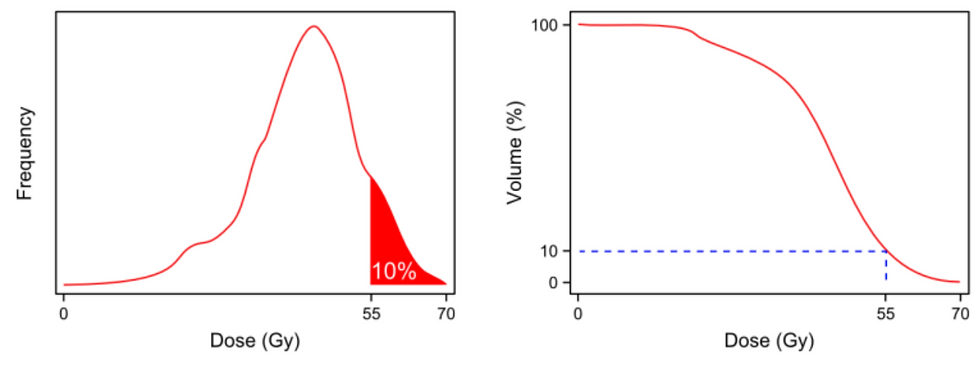top of page

# Understanding the Meaning of DVH Metrics

## The Dose-Volume Histogram

The dose-volume histogram (DVH) is a graphical representation of the distribution of dose within a structure. Here's an example of a differential DVH:The DVH can be used to answer questions like "What is the percent volume that receives 40 Gy or more?" This volume is represented by the shaded area in the following DVH:In order to calculate this area, one can use the cumulative DVH. The cumulative DVH graphs the volume (in the y-axis) that receives the corresponding dose or more in the x-axis.

For example, let's say that 75% of the volume receives 40 Gy or more. Here's what this looks like in both differential and cumulative DVHs:With a cumulative DVH, it's easy to look up the volume for other dose limits. Here's the volume (10%) that receives 55 Gy or more, again in both differential and cumulative DVHs:The cumulative DVH can be used to derive other useful metrics, some of which I explain below. The following metrics use the standard nomenclature developed by the AAPM Task Group 263.

## DVH Metrics

The question I posed above,

What is the percent volume that receives 40 Gy or more?

can be written as V40Gy[%]. The dose may be specified as a percent, relative to a reference dose, and the desired volume may be specified in absolute units (cc). Therefore, other variations of this metric are V40Gy[cc], V40%[%], and V40%[cc].

If V40Gy[%] = 75%, this may be phrased as, "75% of the volume receives 40 Gy or more," or "75% of the volume receives at least 40 Gy."

The question,

What is the least dose that the hottest 75% of the volume receives?

can be written as D75%[Gy]. This is the reverse of the previous question, but here you want to know the dose, given the volume. Just as the previous metric, the units may be in absolute or relative units.

If D75%[Gy] = 40 Gy, it means that "at least 40 Gy is delivered to the hottest 75% of the volume." The word "hottest" just emphasizes that all of the 75% of the volume receives 40 Gy or more.

D0.01cc[Gy] is a useful special-case of this metric. It means "the least dose that the hottest 0.01 cc receives." This is equivalent to the near-maximum dose. The absolute maximum is not always desired because there may be outlier voxels with high doses. (The 0.01 cc value is an example—you may instead be interested in D0.03cc[Gy] or D2%[Gy].)

Similarly, D98%[Gy] is the near-minimum dose.

The question,

What is the percent volume that receives 40 Gy or less?

can be written as CV40Gy[%]. The "C" here stands for "cold" because the volume we're asking about receives less than the specified dose. We can visualize this volume in the following differential DVH:Similarly, the metric DC25%[Gy] represents the question,

What is the highest dose that the coldest 25% of the volume receives?

There are additional metrics that are statistical, such as the mean dose, the median dose, or the standard deviation of the dose. The median dose is actually equivalent to D50%[Gy].

There are also radiobiological metrics, such as the generalized equivalent uniform dose (gEUD) and the normal tissue complication probability (NTCP). I won't discuss these in this blog post.

## Summary

The following table summarizes the metrics I discussed above:

Metric

V40Gy[%]

​What is the percent volume that receives 40 Gy or more?

D75%[Gy]

What is the least dose that the hottest 75% of the volume receives?

D0.01cc[Gy]

What is the near-maximum dose (within 0.01 cc)?

D98%[Gy]

What is the near-minimum dose (within 2% of the volume)?

CV40Gy[%]

What is the percent volume that receives 40 Gy or less?

DC25%[Gy]

What is the highest dose that the coldest 25% of the volume receives?

## Related Posts

See All

A few months ago, I introduced ESAPI Essentials—a toolkit for working with ESAPI (available via NuGet). I've recently added one major feature: asynchronous access to ESAPI for binary plugin scripts. Y

A few months ago, Matt Schmidt started the ESAPI subreddit. It's another useful resource for finding and discussing ESAPI-related topics. According to the description, This community is to post and di

If, for whatever reason, you need to dump out all of the data for a patient from ESAPI, there's a quick and dirty way to do it. Well, there are probably several ways to do it, but here's one I've foun

bottom of page